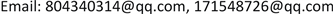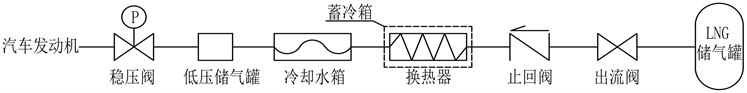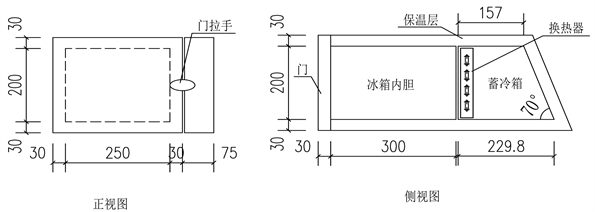﻿ LNG汽车零能耗车载冰箱的理论与实验研究 Theoretical and Experimental Research on LNG Zero Energy Vehicle Refrigerator

Journal of Low Carbon Economy
Vol.07 No.02(2018), Article ID:25126,7 pages
10.12677/JLCE.2018.72007

Theoretical and Experimental Research on LNG Zero Energy Vehicle Refrigerator

Shuyi Chen, Gang Chen, Xinran Ge, Wei Cui

College of Civil Engineering, University of South China, Hengyang HunanReceived: May 8th, 2018; accepted: May 22nd, 2018; published: May 29th, 2018ABSTRACT

In order to protect environment, traditional fuel car will be replaced by new energy car. LNG is one of the new energy that be used widely now. On the other hand, vehicle refrigerator is being used diffusely. Traditional vehicle refrigerator needs automobile engine to provide extra electric energy to support its work. Indirectly, it will consume more fuel. According to the circumstance that car works, we study basic theory and experiment about recover the cold energy that LNG release during the gasification process to cool the refrigerator and provide theoretical direction to invent LNG zero energy vehicle refrigerator.

Keywords:LNG, Car, Cold Energy, Vehicle Refrigerator

LNG汽车零能耗车载冰箱的理论与实验研究1. 引言

2. 回收气化冷能用于车载冰箱的基本原理

2.1. 改造后LNG汽车燃料流程

LNG的使用流程如图1所示。

LNG储存于储罐中，使用时−162℃的LNG从打开的出液阀中流出，经止回阀流入到蓄冷箱中的换热器，在换热器中吸收热量后进入到汽车冷却水箱进行二次换热，气化成5℃~10℃的气态天然气，然后进入低压储气罐，通过稳压阀控制天然气压力使之符合发动机的需要后，向发动机供气  。

2.2. LNG车载冰箱换热器与蓄冷箱工作原理Figure 1. Theory of recycling cold energy to cooling vehicle refrigerator

3. 理论研究

3.1. 冰箱的有效容积与结构形式

3.2. 自驾游汽车日消耗LNG量

LNG必须经气化后才能被汽车发动机使用，资料表明，1 m3 LNG气化后的气态天然气体积约为650 m3  ，则自驾游汽车日消耗LNG量可按下式计算：

${V}_{LNG}=l÷{l}^{\prime }÷650$ (1)

VLNG——自驾游汽车每天消耗的LNG量(m3)。

l——自驾游汽车每天行驶的里程数(km)；据统计，每天行驶的距离平均为300 km。

l'——单位气态天然气能支持汽车行驶的距离(km)；一般为11~14 km (取平均值12.5 km)。

3.3. 日消耗LNG释放气化冷能计算

$Q={V}_{LNG}\cdot \rho \cdot r$ (2)

Q——汽车每天消耗LNG所释放的气化冷能(kJ)。

ρ——LNG的密度(kg/m3)，正常状态下，取ρ = 426

r——单位重量LNG气化所释放的冷能(kJ)，包括汽化潜热和温差吸热两部分，r = 840 kJ   。

3.4. 冰箱单位时间耗冷量计算

${Q}_{X}=i\cdot cop$ (3)

${Q}_{X}$ ——每小时冰箱所消耗的冷量(kJ)。

i——冰箱一天平均耗电量(kW∙h)；目前普通15 L车载冰箱24小时平均耗电量约为1.0 KW∙h。

cop——车载冰箱的能效比，cop = 2.6  。

${Q}_{X}=\left(1.0×2.6÷24\right)×3.6×{10}^{3}=390\left(kJ\right)$

3.5. 蓄冷箱蓄冷量计算

${Q}_{C}={Q}_{X}÷{\eta }_{1}÷{\eta }_{2}÷{\eta }_{3}$ (3)

QC——保证汽车停驶后车载冰箱正常工作所需的需冷量(kJ)。

${\eta }_{1}$ ——蓄冷效率， ${\eta }_{1}=80%$

${\eta }_{2}$ ——释冷效率， ${\eta }_{2}=80%$

${\eta }_{3}$ ——保冷效率， ${\eta }_{3}=60%$

3.6. 蓄冷材料选择与使用量计算

$4062.5÷335=12.13\left(kg\right)$

3.7. 换热器的选择与换热面积设计

3.7.1. 换热器选型

3.7.2. 换热器设计

$Q={\int }_{0}^{F}K\Delta t\text{d}F+{W}_{LNG}\cdot {c}_{p}\cdot \gamma$ (4)Figure 2. Theory of heat exchanger

$K=\frac{1}{\left(\frac{{d}_{0}}{{d}_{i}}\right)\frac{1}{{h}_{i}}+\left(\frac{{d}_{0}}{{d}_{i}}\right){R}_{f,i}+\frac{{d}_{0}}{2\lambda }\mathrm{ln}\left(\frac{{d}_{0}}{{d}_{i}}\right)+{R}_{f,0}+\frac{1}{{h}_{0}}}$ (5)

${\eta }_{0}=1-\frac{{F}_{f}}{F}\left(1-{\eta }_{f}\right)$ (6)

Q——LNG在换热器中吸收的热量(W)；

K——换热器的总传热系数[W/(m2∙℃)]；

F——换热器的总换热面积(m2)； $F={F}_{f}+{F}_{t}$

Δt——LNG气化成CNG后平均温差(℃)；

WLNG——LNG的质量流量(kg/s)；

${c}_{p}$ ——LNG的定压比热[kJ/(kg∙℃)]；

$\gamma$ ——LNG的汽化潜热(kJ/kg)；

${\eta }_{f}$ ——翅片的换热效率，

${\eta }_{f}=\frac{\text{tanh}\left({W}_{LNG}r\phi \right)}{{W}_{LNG}r\phi }$ (7)

${F}_{f}$ ——翅片换热面积(m2)；

${F}_{f}=2{n}_{f}\left[H×W-{N}_{t}{n}_{t}\text{π}{\left(\frac{{d}_{0}}{2}\right)}^{2}\right]$ (8)

${F}_{t}$ ——换热管换热面积(m2)；

${F}_{t}={N}_{t}{n}_{t}\text{π}{d}_{0}\left(L-{\delta }_{f}{n}_{f}\right)$ (9)

nf——翅片数；

nt——每排的热管数；

Nt——换热管的排数，本文取值为1；

r——换热管的半径(m)；

$\phi$ ——粘度修正系数。

d0、h0、Rf,0、di、hi、Rf,i分别是换热管内径、管内流体对流换热系数、管内污垢热阻、管外径、管外流体对流换热系数、管内污垢热阻；H、W、L分别表示换热器的长度、宽度和高度。

3.8. 蓄冷箱体积计算Figure 3. Structure size and shape of the refrigerator. (a) Front view of the refrigerator; (b) The strakes of the refrigeratorTable 1. The temperature of monitoring point/˚C

4. 实验

5. 结论

LNG气化冷能除了用于冰箱外，尚有大部分冷能用于了冷却水箱。如果车尾箱空间允许，可以支持更大容量的冰箱工作。

Theoretical and Experimental Research on LNG Zero Energy Vehicle Refrigerator[J]. 低碳经济, 2018, 07(02): 59-65. https://doi.org/10.12677/JLCE.2018.72007

1. 1. 晓青. 绿色环保拉动燃气汽车的未来市场[J]. 市场研究, 2010, 30(3): 19-24.

2. 2. 王凌士, 张学军, 王晓蕾, 王炜. LNG冷能用于气体直接接触法制取冰浆研究[J]. 低温工程, 2012, 186(2): 26-27.

3. 3. 高春梅, 李清, 邵震宇. 液化天然气储存及应用技术[J]. 城市燃气, 2002, 324(2): 18-22.

4. 4. 王强. 绿色汽车燃料–液化天然气冷量回收汽车空调系统研究[D]: [博士学位论文]. 西安: 西安交通大学, 2003.

5. 5. 冯陈玥, 段兆芳, 单卫国. LNG汽车发展现状及相关问题分析[J]. 中国能源, 2014, 36(2): 32-35.

6. 6. 汪磊. LNG汽车燃料系统改装与冷能回收利用探析[J]. 物流工程与管理, 2015, 37(7): 237-238.

7. 7. 王方, 付一珂, 范晓伟, 朱彩霞. 液化天然气(LNG)冷能利用研究进展[J]. 化工进展, 2016, 35(3): 748-753.

8. 8. 金树峰. LNG汽车燃料系统与空调冷能利用[D]: [硕士学位论文]. 兰州: 兰州理工大学, 2014.

9. 9. 张立超, 刘怡君. 低碳交通视角下的LNG汽车产业现状与前景预测[J]. 中国软科学, 2014(5): 66-75.

10. 10. 袁帅. 基于人性化设计的车载冰箱设计研究[D]: [硕士学位论文]. 秦皇岛: 燕山大学, 2015.

11. 11. 吴北民, 陈叔平, 殷劲松, 谢福寿, 姚淑婷. 车用液化天然气供气系统工艺与设备[J]. 煤气与热力, 2012, 2(32): B9-B12.

12. 12. 张祁. 全球LNG市场分析和对中国LNG市场的展望与建议[J]. 城市燃气, 2005(4): 19-24.

13. 13. 单程程. LNG冷能综合利用规划方案评价研究[D]: [硕士学位论文]. 大连: 大连理工大学, 2016.

14. 14. 陈叔平, 谢高峰, 李秋英, 昌锟. LNG、L-CNG、CNG加气站的比较[J]. 煤气与热力, 2007, 27(7): 27-30.

15. 15. 杨颖, 沈海英. 复合低温相变蓄冷材料的实验研究[J]. 低温物理学报, 2009, 31(2): 144-147.

16. 16. 连之伟. 热质交换原理与设备[M]. 第三版. 北京: 中国建筑工业出版社, 2012.

17. 17. 薛提微. 换热器的热力设计方法与性能评价[D]: [硕士学位论文]. 济南: 山东大学, 2017.